November 23, 2021

# Class 10 Maths Chapter 2 Polynomials Complete Study Material

In class 10 maths chapter 2 polynomials, as the name suggests we are going to study the polynomials. We are familiar with the term polynomials as we have already studied polynomials in the previous class. In class 9th NCERT we read and solve problems related to the degree of the polynomial. We studied in detail polynomials in one variable and their degrees.

It’s perfectly normal to forget things, especially when you have not been in touch for a long time. So, to make sure your basics for this chapter are clear, we have written a short summary of all the important points that we studied in class IXth. If you don’t remember anything even after looking at these points, then it’s recommended to revisit chapter 2 of class 9th maths before proceeding with this chapter.

## Class 10 Maths Chapter 2 Polynomials Complete Study Material

So, before we go on discussing chapter 2 of class Xth, let’s first make sure we understand important terms and topics regarding this chapter from class 9th.

What is a polynomial?

Polynomials are simply algebraic expressions that have variable, coefficient, and constant.

e.g: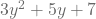The word polynomial comes from the Greek language. In Greek, the word ‘poly’ means ‘many’, and ‘nomial’ means ‘term’, together these two words make ‘polynomial’ or ‘many terms’ in English.

what is a ‘term’ in a polynomial?

A term in a polynomial can be the product of a number and 1 or more variables with exponents or it can simply be variable or constant.

e.g in the polynomial equation, {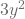,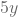&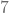} are all terms.

What is a ‘coefficient’ in a polynomial?

A coefficient is an integer that multiplies with a variable of a single term. e.g in the above polynomial, 3 is the coefficient of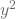, 2 is the coefficient of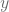, and 7 is the coefficient of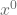(= 1).

What is the ‘Degree of polynomial’?

In a polynomial expression, the variable with the highest power is called the degree of that polynomial. e.g in the equation, 2 is the highest power, therefore 2 is the degree of this polynomial expression.

Here are some more important terms:

• A polynomial that has 1, 2, and 3 is called monomial, binomial, and trinomial respectively.
• The polynomial with degrees 1, or 2, or 3 are called linear, quadratic, and cubic polynomials respectively.
•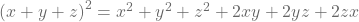•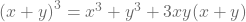•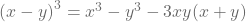•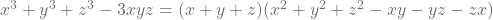### Class 10 Maths Chapter 2 Polynomials Explanation – part 1

Now that we know all the important terms from the previous class, we can begin our explanation for class 10 maths chapter 2 polynomials.

What is ‘Zeroes of polynomial’?

For a polynomial, there exist some values of the variable for which the given polynomial will be 0, these values are considered as ‘zeroes of polynomial’.

There could be some values of the variable for which the polynomial will be zero. These values are called zeros of a polynomial. In simple words, any value putting which the whole polynomial expression will become zero is called zero of that polynomial.

# Geometrical Meaning of the Zeroes of a Polynomial

In earlier classes we learned how we can find out the zeroes of a polynomial, now in this section of the chapter, we will learn to find the zeroes of different polynomials on a graph.

# Relationship between Zeroes and Coefficients of a Polynomial

In this section, we will learn the relationship between the zeroes and coefficients of a polynomial. One can easily determine the number of zeroes in a polynomial just by looking at its degree, because, number of zeroes of a polynomial is equal to the degree of the polynomial.

The relationship between the zeroes and coefficients of a quadratic polynomial is expressed as:

•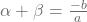•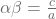Or, we can say that

•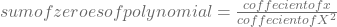•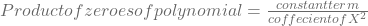The relationship between the zeroes and coefficients of a Cubic polynomial is expressed as:

•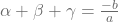,
•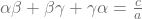,
•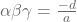#### Class 10 Maths Chapter 2 Polynomials Explanation – part 2

So far in this chapter, we have covered almost all important concepts that we are to learn in class 10th, now let’s move on to the final section of this chapter and complete it as well.

# Division Algorithm for Polynomials

According to the division algorithm of polynomial, if there are two polynomilas say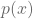and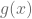(whereis not equal to 0), then the division of these polynomials can be wrtten as: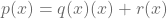{here,is dividend,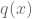is quotient,is divisor, &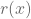is remainder}

This expression is same as the euclids division lemma i.e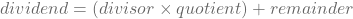With this, we have completed all the topics in class 10 maths chapter 2 polynomials. now, let’s see the blueprint of this chapter so that we know how much time should be given to this lesson.

Maths Chapter 2 Blueprint For class 10 (Polynomials)
1 Marker 1 question
2 Marker 1 question
4 marker 1 question
Total questions 3
Total marks 7

Note: The above marking scheme is set according to the sample released by CBSE this year, it is subjected to change, and the number of questions and marks allotted to them may change in the board exam. Students are advised to be prepared for all situations.

From this chapter one can expect a total of 3 questions worth 7 marks. There will be 1 question each from 1 marker, 2 markers, and 4 markers. Make sure to practice the optional exercise given in the NCERT book, for your convenience, we have given the NCERT solutions for chapter 2 polynomials, but, before you look at the solutions, make sure to try them by yourself first.